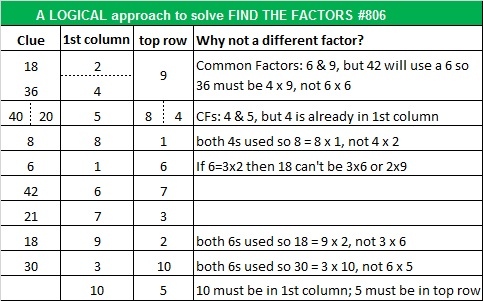# 806 and a Level 6 Dunce Cap?

When I put this post together I took a second look at today’s puzzle and thought, “That looks a little like a dunce cap.” That thought led me to two very interesting articles whose information surprised me greatly.

The first one titled “The Dunce Cap Wasn’t Always so Stupid” explains that long ago when the dunce cap was first introduced by the brilliant Scotsman John Duns Scotus, it became a symbol of exceptional intellect. In fact wizard hats were most likely modeled after them. Unfortunately, this positive perception of the caps remained for only a couple of centuries.

The second article is short but helped me visualize Topology’s Dunce Hat. I enjoyed watching the animation of this mathematical concept.

I hope you will enjoy trying to solve the puzzle. It is a Level 6, so it won’t be easy. If you succeed, you’ll deserve to feel that you have exceptional intellect.Print the puzzles or type the solution on this excel file: 10-factors 801-806

Now here is some information about the number 806:

• 806 is a composite number.
• Prime factorization: 806 = 2 x 13 x 31
• The exponents in the prime factorization are 1, 1, and 1. Adding one to each and multiplying we get (1 + 1)(1 + 1)(1 + 1) = 2 x 2 x 2 = 8. Therefore 806 has exactly 8 factors.
• Factors of 806: 1, 2, 13, 26, 31, 62, 403, 806
• Factor pairs: 806 = 1 x 806, 2 x 403, 13 x 62, or 26 x 31
• 806 has no square factors that allow its square root to be simplified. √806 ≈ 28.390139.806 is a palindrome in three different bases:

• 11211 BASE 5 because 1(625) + 1(125) + 2(25) + 1(5) + 1(1) = 806
• 1C1 BASE 23 (C is 12 base 10) because 1(23²) + 12(23) + 1(1) = 806
• QQ BASE 30 (Q is 26 base 10) because 26(30) + 26(1) = 806, which follows naturally from the fact that 26 × 31 = 806

806 is the hypotenuse of Pythagorean triple 310-744-806 which is 5-12-13 times 62.

And 806 can be written as the sum of three squares seven different ways:

• 26² + 11² + 3² = 806
• 26² + 9² + 7² = 806
• 25² + 10² + 9² = 806
• 23² + 14² + 9² = 806
• 21² + 19² + 2² = 806
• 21² + 14² + 13² = 806
• 19² + 18² + 11² = 8061.Steve Morris
•ivasallay